Function Repository Resource:

# OrthogonalPolynomialVandermondeSolve

Solve an orthogonal polynomial Vandermonde linear system

Contributed by: Jan Mangaldan
 ResourceFunction["OrthogonalPolynomialVandermondeSolve"][poly,{a1,a2,…},{b1,b2,…}] solves the primal Vandermonde problem V.x==b, where V(a1,a2,…) is the orthogonal polynomial Vandermonde matrix with respect to the basis represented by poly.

## Details and Options

The argument poly can be any of the following:
 "ChebyshevFirst" Chebyshev polynomial of the first kind ChebyshevT[i,x] "ChebyshevSecond" Chebyshev polynomial of the second kind ChebyshevU[i,x] "Hermite" Hermite polynomial HermiteH[i,x] "Laguerre" Laguerre polynomial LaguerreL[i,x] "Legendre" Legendre polynomial LegendreP[i,x] {"Gebenbauer",m} Gegenbauer polynomial GegenbauerC[i,m,x] {"Laguerre",a} associated Laguerre polynomial LaguerreL[i,a,x] {"Jacobi",a,b} Jacobi polynomial JacobiP[i,a,b,x]
Strings in specifications like "Legendre" and {"Jacobi",a,b} can be replaced with the corresponding symbols, as in LegendreP and {JacobiP,a,b}.
If the argument poly is set to "Monomial", the classical Vandermonde solution is generated.
ResourceFunction["OrthogonalPolynomialVandermondeSolve"][poly,{a1,a2,},{b1,b2,},"Transpose"True] solves the dual Vandermonde problem Transpose[V].x==b.

## Examples

### Basic Examples (1)

Solve a Chebyshev–Vandermonde system:

 In:=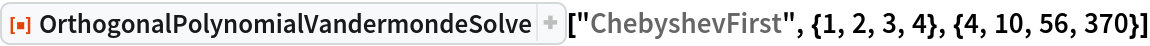Out=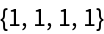### Scope (2)

Solve a Jacobi–Vandermonde system with symbolic parameters and vectors:

 In:=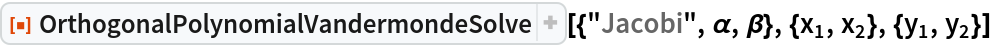Out=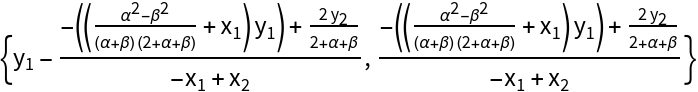An equivalent specification:

 In:=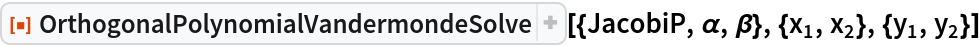Out=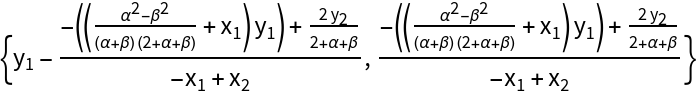Solve a Hermite–Vandermonde system with numeric vectors:

 In:=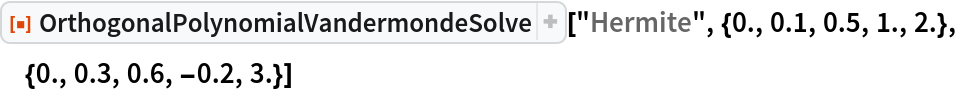Out=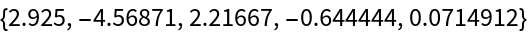### Options (2)

#### Transpose (2)

With "Transpose"False, OrthogonalPolynomialVandermondeSolve solves a primal Vandermonde problem:

 In:=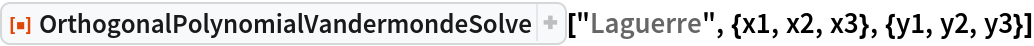Out=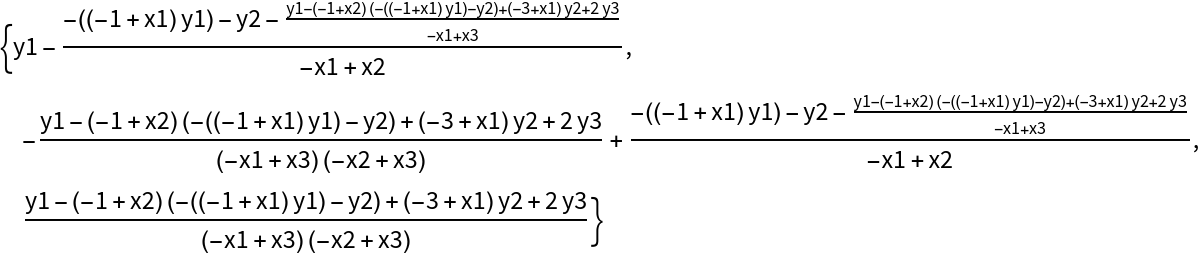In:=Out=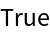With "Transpose"True, OrthogonalPolynomialVandermondeSolve solves a dual Vandermonde problem:

 In:=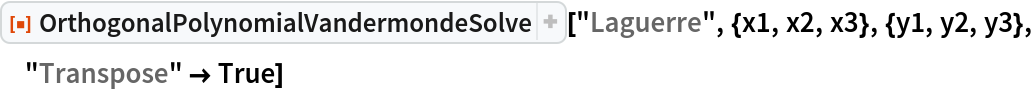Out=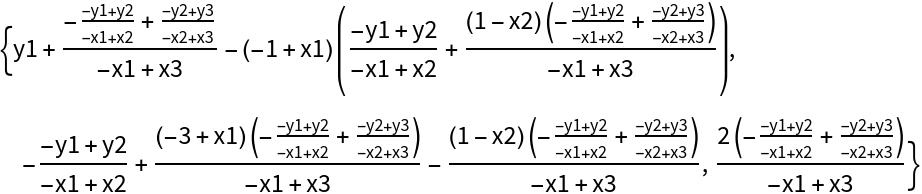In:=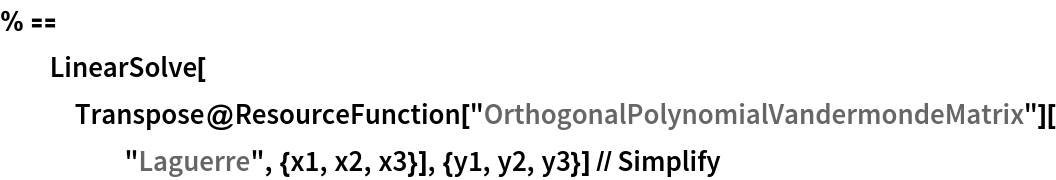Out=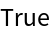### Applications (2)

Get the Legendre series coefficients of an interpolating polynomial:

 In:=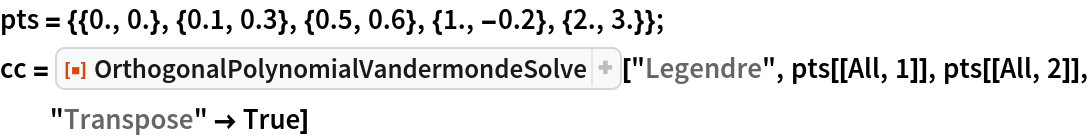Out=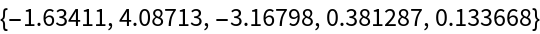Use the resource function OrthogonalPolynomialSum to get the corresponding orthogonal polynomial series, and compare with the result of InterpolatingPolynomial:

 In:=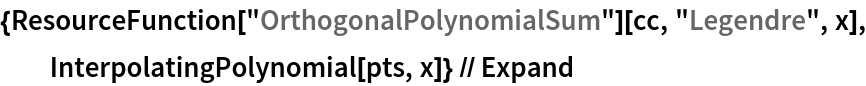Out=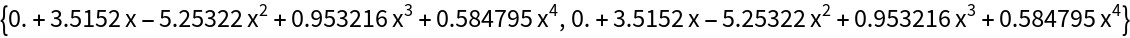### Properties and Relations (2)

OrthogonalPolynomialVandermondeSolve is more efficient than using LinearSolve on an orthogonal polynomial Vandermonde system:

 In:=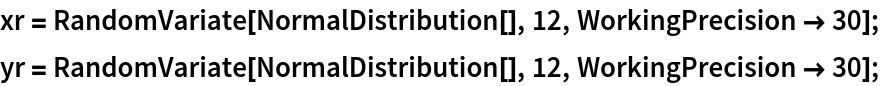In:=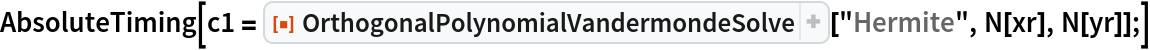Out=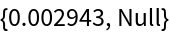In:=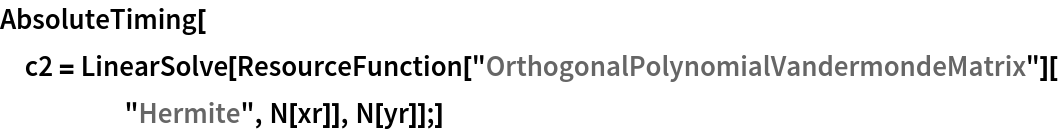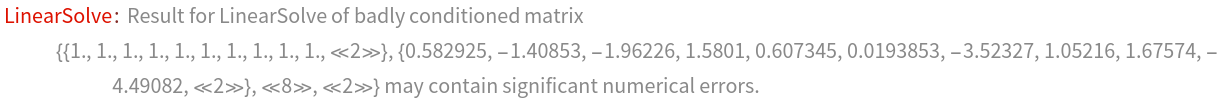Out=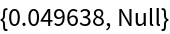The result of OrthogonalPolynomialVandermondeSolve is also often more accurate:

 In:=In:=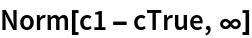Out=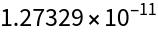In:=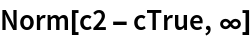Out=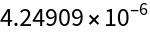## Version History

• 1.0.0 – 27 April 2021